|

# 2018·BIFF丨遇见智能时代,定义生活新方式

现代科技充斥着生活的各个角落，人们的吃穿住行越来越智能化，就像电影《机器人总动员》描述的一样，科技接连不断地为我们的生活创造惊喜，人们的生活方式也越来越趋于个性化、自动化和趣味化。随着社会经济水平的提升，人性化的家居生活、智能化不再是富豪达人的专属，智能电子技术、计算机网络与通讯技术的应用，正在给人们的家居生活带来全新的体验，家居智能化已经成为一种趋势。

很多消费者迫切的想知道属于自己的“未来之家”究竟长什么样子？6月14日-17日，来北京国际家居展暨智能生活节（以下简称“2018BIFF”），邂逅未来的家，解锁智能生活新方式。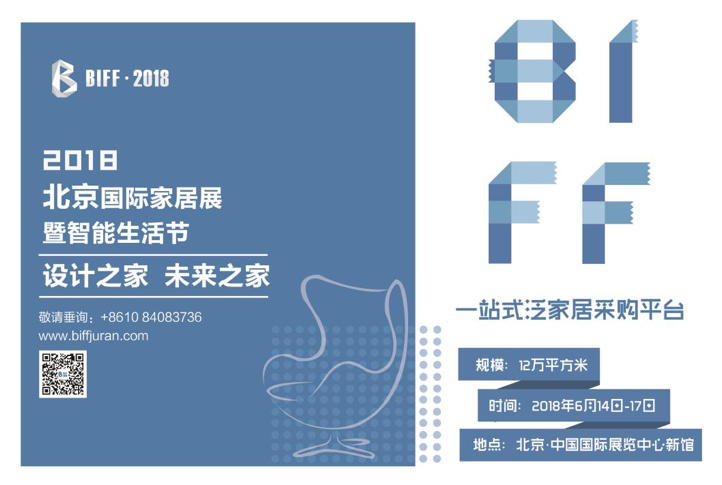2018 BIFF由北京居然之家投资控股集团有限公司、中国国际展览中心集团公司联合主办，北京中展居然国际展览有限公司承办。本次家居展延续了第一届“设计之家，未来之家”的主题，通过科技赋予家居生活智能化新体验。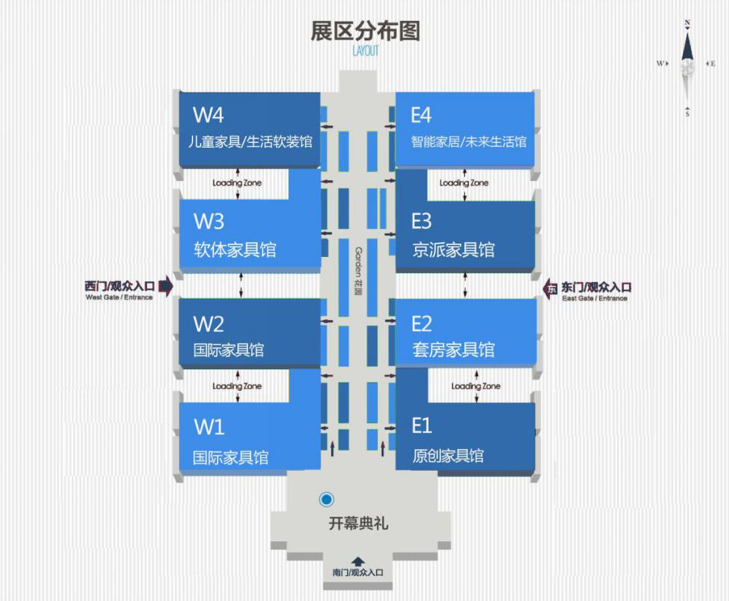2018 BIFF将推出八个展区，包括国际家具馆、软体家具馆、儿童家具/生活软装馆、原创家具馆、套房家具馆、京派家具馆和智能家居/未来生活馆。其中，位于E4位置的智能家居/未来生活馆展区集科技与创意为一体，带你体验超出想象的“黑科技”家居生活。

未来生活创意馆全景展示了属于未来的智能家居创意，给人以巨大的想象空间。无人机、智能家电、VR全景、3D打印技术等生活概念的展示，重新定义衣食住行。随处可见的科技因素不禁让人幻想未来生活的美好，致力于让生活更加舒适、健康和便捷。综合来看，本届北京国际家居展的未来生活创意馆主要有以下三大看点。

智能黑科技，解锁互动新姿势

在首届北京国际家居展上，未来生活及智能家居的参展品占比总体的25%，创意、科技、智能、便捷、品质成为大家热议的话题。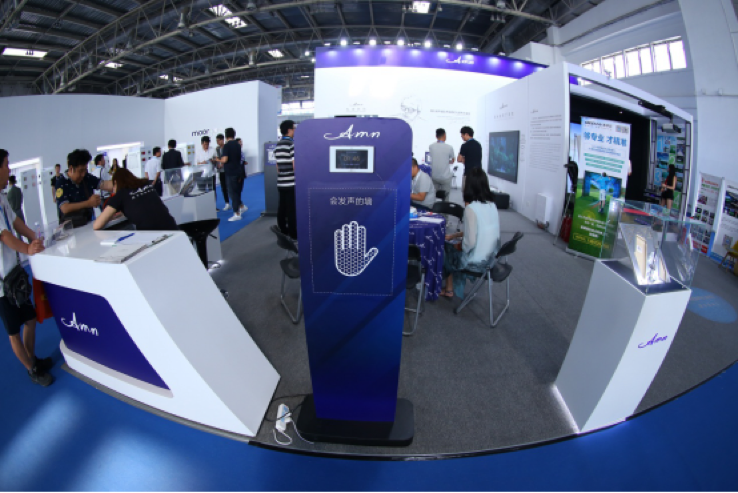2017BIFF参展智能产品

在E4智能家居/未来生活馆里，国内外科技行业知名企业同场“竞技”，上演无比精彩的科技盛宴。

中关村在线作为国内为数不多的体验馆全产业链综合服务商，为迎接科技发烧友的到来，也做了充足的准备。不仅让现场观众看到中关村在线在现场打造的未来之家，从策划、设计、研发、施工、运营管理等方面的综合服务水平，更是打造了让观众近距离体验融合视觉、触觉、听觉等全方位的沉浸式空间体验。

阿米纳在本次未来生活创意馆上惊喜亮相，通过三维立体影视内容，以及与影片内容一致的全方位立体声效，给予观众全方位的感官冲击，创造出身临其境的沉浸体验。除此之外，第二届北京国际家居展还与轻奢类企业携手，在现场共同打造“以理想生活方式”活动呈现给大家，最潮流、最前沿、最in好物汇集为展览的一大“奇观”。

据悉，即将举办的第二届北京国际家居展将融教育娱乐于一体，既有极具科技感的无人超市、无人机、自动驾驶技术，又有生活感十足的电竞娱乐、时尚设计、城市运动、亲子教育等内容，展会现场亦将被装饰为科技感、时尚感、奢华感爆棚的“未来小镇”，供与会者参观体验，解锁人机互动新模式。

创意家居，打造轻奢生活方式

随着大众消费理念的不断升级，当代居民对品质生活的需求越来越高，“轻奢华、新时尚”的理念被越来越多的人奉为生活标签。现代人多元化的生活方式，促使传统家居行业也开始紧急革新突破、提升标准，“创意智能家居”便是家居行业当下转型的主要方向之一。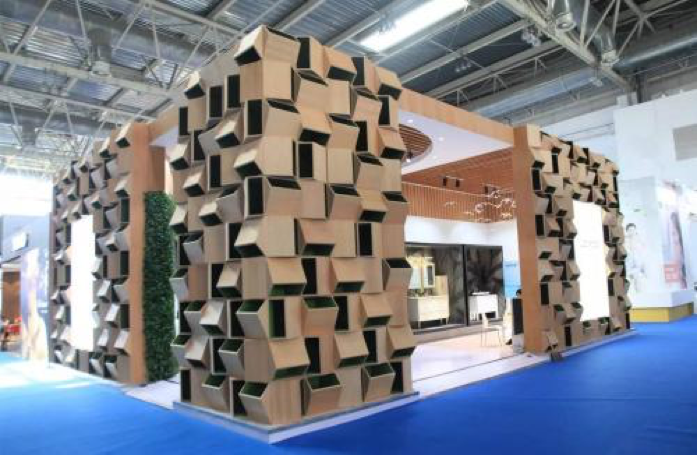在2018年北京国际家居展上，中关村在线倾力诠释“未来之家”，寺库将展区主题定位“理想生活方式”体验区，此外，哈曼、阿米纳、软银、奇弩、SONOS、优必选、阿斯蒙迪、稀奇艺术、森贝尔以及Boyteks等众多品牌携手打造未来生活空间，定义了以”轻奢“为主题的未来生活方式。

2018 BIFF顺应市场趋势，推出“智能家居/未来生活馆”，一站式亲子、美食、娱乐“生活趴”，打造顶级消费体验区。届时，“未来之家”高峰论坛也将如期举行，汇集行业精英，探讨创意家居对生活方式的颠覆，发掘未来生活的无限可能。

独家高端客源，引领消费升级

家居行业的转型及消费升级业已到来。2017BIFF的成功举办，推动了家居行业向智能化的转型升级，未来随着科技的进步与发展，设计加工的成本会越来越低，消费者越来越亲近智能家居，间接推动消费升级。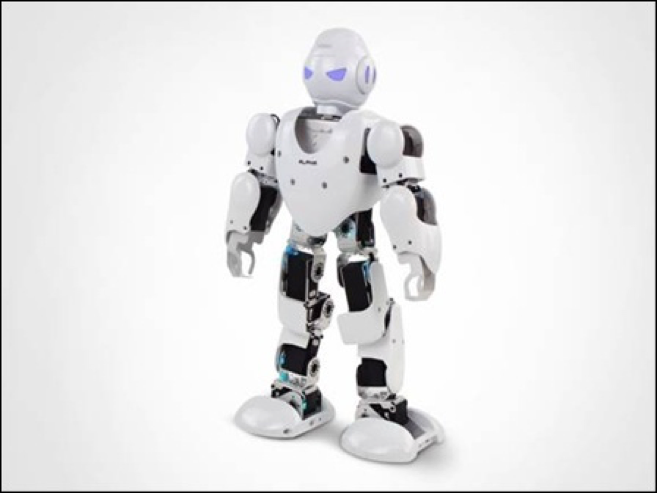作为本次展会的主办方之一，居然之家在全国拥有200多家分店，20多个顶层设计中心，范围覆盖10万经销商、10万设计师，且核心客户聚焦于一线城市年收入20万以上的年轻化中高端消费人群。而这一群体正好是科技智能产品的目标用户，他们需要充满科技感、设计感、高品质的产品和服务，在这里，高端人群的消费潜力一触即发。据悉，第一届家居展上“优必选机器人”、“进口商务车”等众多企业都收获了丰厚的订单，而2018BIFF预计到场消费者更可突破20万人次，它所带来的购买力，更值得我们期待。

更多精彩，6月14-17日，2018北京国际家居展，等你来发现！

`声明：本文由入驻焦点开放平台的作者撰写，除焦点官方账号外，观点仅代表作者本人，不代表焦点立场错误信息举报电话： 400-099-0099，邮箱：jubao@vip.sohu.com，或点此进行意见反馈，或点此进行举报投诉。`A B C D E F G H J K L M N P Q R S T W X Y Z
A - B - C - D - E
• A
• 鞍山
• 安庆
• 安阳
• 安顺
• 安康
• 澳门
• B
• 北京
• 保定
• 包头
• 巴彦淖尔
• 本溪
• 蚌埠
• 亳州
• 滨州
• 北海
• 百色
• 巴中
• 毕节
• 保山
• 宝鸡
• 白银
• 巴州
• C
• 承德
• 沧州
• 长治
• 赤峰
• 朝阳
• 长春
• 常州
• 滁州
• 池州
• 长沙
• 常德
• 郴州
• 潮州
• 崇左
• 重庆
• 成都
• 楚雄
• 昌都
• 慈溪
• 常熟
• D
• 大同
• 大连
• 丹东
• 大庆
• 东营
• 德州
• 东莞
• 德阳
• 达州
• 大理
• 德宏
• 定西
• 儋州
• 东平
• E
• 鄂尔多斯
• 鄂州
• 恩施
F - G - H - I - J
• F
• 抚顺
• 阜新
• 阜阳
• 福州
• 抚州
• 佛山
• 防城港
• G
• 赣州
• 广州
• 桂林
• 贵港
• 广元
• 广安
• 贵阳
• 固原
• H
• 邯郸
• 衡水
• 呼和浩特
• 呼伦贝尔
• 葫芦岛
• 哈尔滨
• 黑河
• 淮安
• 杭州
• 湖州
• 合肥
• 淮南
• 淮北
• 黄山
• 菏泽
• 鹤壁
• 黄石
• 黄冈
• 衡阳
• 怀化
• 惠州
• 河源
• 贺州
• 河池
• 海口
• 红河
• 汉中
• 海东
• I
• J
• 晋中
• 锦州
• 吉林
• 鸡西
• 佳木斯
• 嘉兴
• 金华
• 景德镇
• 九江
• 吉安
• 济南
• 济宁
• 焦作
• 荆门
• 荆州
• 江门
• 揭阳
• 金昌
• 酒泉
• 嘉峪关
K - L - M - N - P
• K
• 开封
• 昆明
• 昆山
• L
• 廊坊
• 临汾
• 辽阳
• 连云港
• 丽水
• 六安
• 龙岩
• 莱芜
• 临沂
• 聊城
• 洛阳
• 漯河
• 娄底
• 柳州
• 来宾
• 泸州
• 乐山
• 六盘水
• 丽江
• 临沧
• 拉萨
• 林芝
• 兰州
• 陇南
• M
• 牡丹江
• 马鞍山
• 茂名
• 梅州
• 绵阳
• 眉山
• N
• 南京
• 南通
• 宁波
• 南平
• 宁德
• 南昌
• 南阳
• 南宁
• 内江
• 南充
• P
• 盘锦
• 莆田
• 平顶山
• 濮阳
• 攀枝花
• 普洱
• 平凉
Q - R - S - T - W
• Q
• 秦皇岛
• 齐齐哈尔
• 衢州
• 泉州
• 青岛
• 清远
• 钦州
• 黔南
• 曲靖
• 庆阳
• R
• 日照
• 日喀则
• S
• 石家庄
• 沈阳
• 双鸭山
• 绥化
• 上海
• 苏州
• 宿迁
• 绍兴
• 宿州
• 三明
• 上饶
• 三门峡
• 商丘
• 十堰
• 随州
• 邵阳
• 韶关
• 深圳
• 汕头
• 汕尾
• 三亚
• 三沙
• 遂宁
• 山南
• 商洛
• 石嘴山
• T
• 天津
• 唐山
• 太原
• 通辽
• 铁岭
• 泰州
• 台州
• 铜陵
• 泰安
• 铜仁
• 铜川
• 天水
• 天门
• W
• 乌海
• 乌兰察布
• 无锡
• 温州
• 芜湖
• 潍坊
• 威海
• 武汉
• 梧州
• 渭南
• 武威
• 吴忠
• 乌鲁木齐
X - Y - Z
• X
• 邢台
• 徐州
• 宣城
• 厦门
• 新乡
• 许昌
• 信阳
• 襄阳
• 孝感
• 咸宁
• 湘潭
• 湘西
• 西双版纳
• 西安
• 咸阳
• 西宁
• 仙桃
• 西昌
• Y
• 运城
• 营口
• 盐城
• 扬州
• 鹰潭
• 宜春
• 烟台
• 宜昌
• 岳阳
• 益阳
• 永州
• 阳江
• 云浮
• 玉林
• 宜宾
• 雅安
• 玉溪
• 延安
• 榆林
• 银川
• Z
• 张家口
• 镇江
• 舟山
• 漳州
• 淄博
• 枣庄
• 郑州
• 周口
• 驻马店
• 株洲
• 张家界
• 珠海
• 湛江
• 肇庆
• 中山
• 自贡
• 资阳
• 遵义
• 昭通
• 张掖
• 中卫

1室1厅1厨1卫1阳台

1
2
3
4
5

0
1
2

1

1

0
1
2
3报名成功，资料已提交审核A B C D E F G H J K L M N P Q R S T W X Y Z
A - B - C - D - E
• A
• 鞍山
• 安庆
• 安阳
• 安顺
• 安康
• 澳门
• B
• 北京
• 保定
• 包头
• 巴彦淖尔
• 本溪
• 蚌埠
• 亳州
• 滨州
• 北海
• 百色
• 巴中
• 毕节
• 保山
• 宝鸡
• 白银
• 巴州
• C
• 承德
• 沧州
• 长治
• 赤峰
• 朝阳
• 长春
• 常州
• 滁州
• 池州
• 长沙
• 常德
• 郴州
• 潮州
• 崇左
• 重庆
• 成都
• 楚雄
• 昌都
• 慈溪
• 常熟
• D
• 大同
• 大连
• 丹东
• 大庆
• 东营
• 德州
• 东莞
• 德阳
• 达州
• 大理
• 德宏
• 定西
• 儋州
• 东平
• E
• 鄂尔多斯
• 鄂州
• 恩施
F - G - H - I - J
• F
• 抚顺
• 阜新
• 阜阳
• 福州
• 抚州
• 佛山
• 防城港
• G
• 赣州
• 广州
• 桂林
• 贵港
• 广元
• 广安
• 贵阳
• 固原
• H
• 邯郸
• 衡水
• 呼和浩特
• 呼伦贝尔
• 葫芦岛
• 哈尔滨
• 黑河
• 淮安
• 杭州
• 湖州
• 合肥
• 淮南
• 淮北
• 黄山
• 菏泽
• 鹤壁
• 黄石
• 黄冈
• 衡阳
• 怀化
• 惠州
• 河源
• 贺州
• 河池
• 海口
• 红河
• 汉中
• 海东
• I
• J
• 晋中
• 锦州
• 吉林
• 鸡西
• 佳木斯
• 嘉兴
• 金华
• 景德镇
• 九江
• 吉安
• 济南
• 济宁
• 焦作
• 荆门
• 荆州
• 江门
• 揭阳
• 金昌
• 酒泉
• 嘉峪关
K - L - M - N - P
• K
• 开封
• 昆明
• 昆山
• L
• 廊坊
• 临汾
• 辽阳
• 连云港
• 丽水
• 六安
• 龙岩
• 莱芜
• 临沂
• 聊城
• 洛阳
• 漯河
• 娄底
• 柳州
• 来宾
• 泸州
• 乐山
• 六盘水
• 丽江
• 临沧
• 拉萨
• 林芝
• 兰州
• 陇南
• M
• 牡丹江
• 马鞍山
• 茂名
• 梅州
• 绵阳
• 眉山
• N
• 南京
• 南通
• 宁波
• 南平
• 宁德
• 南昌
• 南阳
• 南宁
• 内江
• 南充
• P
• 盘锦
• 莆田
• 平顶山
• 濮阳
• 攀枝花
• 普洱
• 平凉
Q - R - S - T - W
• Q
• 秦皇岛
• 齐齐哈尔
• 衢州
• 泉州
• 青岛
• 清远
• 钦州
• 黔南
• 曲靖
• 庆阳
• R
• 日照
• 日喀则
• S
• 石家庄
• 沈阳
• 双鸭山
• 绥化
• 上海
• 苏州
• 宿迁
• 绍兴
• 宿州
• 三明
• 上饶
• 三门峡
• 商丘
• 十堰
• 随州
• 邵阳
• 韶关
• 深圳
• 汕头
• 汕尾
• 三亚
• 三沙
• 遂宁
• 山南
• 商洛
• 石嘴山
• T
• 天津
• 唐山
• 太原
• 通辽
• 铁岭
• 泰州
• 台州
• 铜陵
• 泰安
• 铜仁
• 铜川
• 天水
• 天门
• W
• 乌海
• 乌兰察布
• 无锡
• 温州
• 芜湖
• 潍坊
• 威海
• 武汉
• 梧州
• 渭南
• 武威
• 吴忠
• 乌鲁木齐
X - Y - Z
• X
• 邢台
• 徐州
• 宣城
• 厦门
• 新乡
• 许昌
• 信阳
• 襄阳
• 孝感
• 咸宁
• 湘潭
• 湘西
• 西双版纳
• 西安
• 咸阳
• 西宁
• 仙桃
• 西昌
• Y
• 运城
• 营口
• 盐城
• 扬州
• 鹰潭
• 宜春
• 烟台
• 宜昌
• 岳阳
• 益阳
• 永州
• 阳江
• 云浮
• 玉林
• 宜宾
• 雅安
• 玉溪
• 延安
• 榆林
• 银川
• Z
• 张家口
• 镇江
• 舟山
• 漳州
• 淄博
• 枣庄
• 郑州
• 周口
• 驻马店
• 株洲
• 张家界
• 珠海
• 湛江
• 肇庆
• 中山
• 自贡
• 资阳
• 遵义
• 昭通
• 张掖
• 中卫• 手机• 分享
• 设计
免费设计
• 计算器
装修计算器
• 入驻
合作入驻
• 联系
联系我们
• 置顶
返回顶部